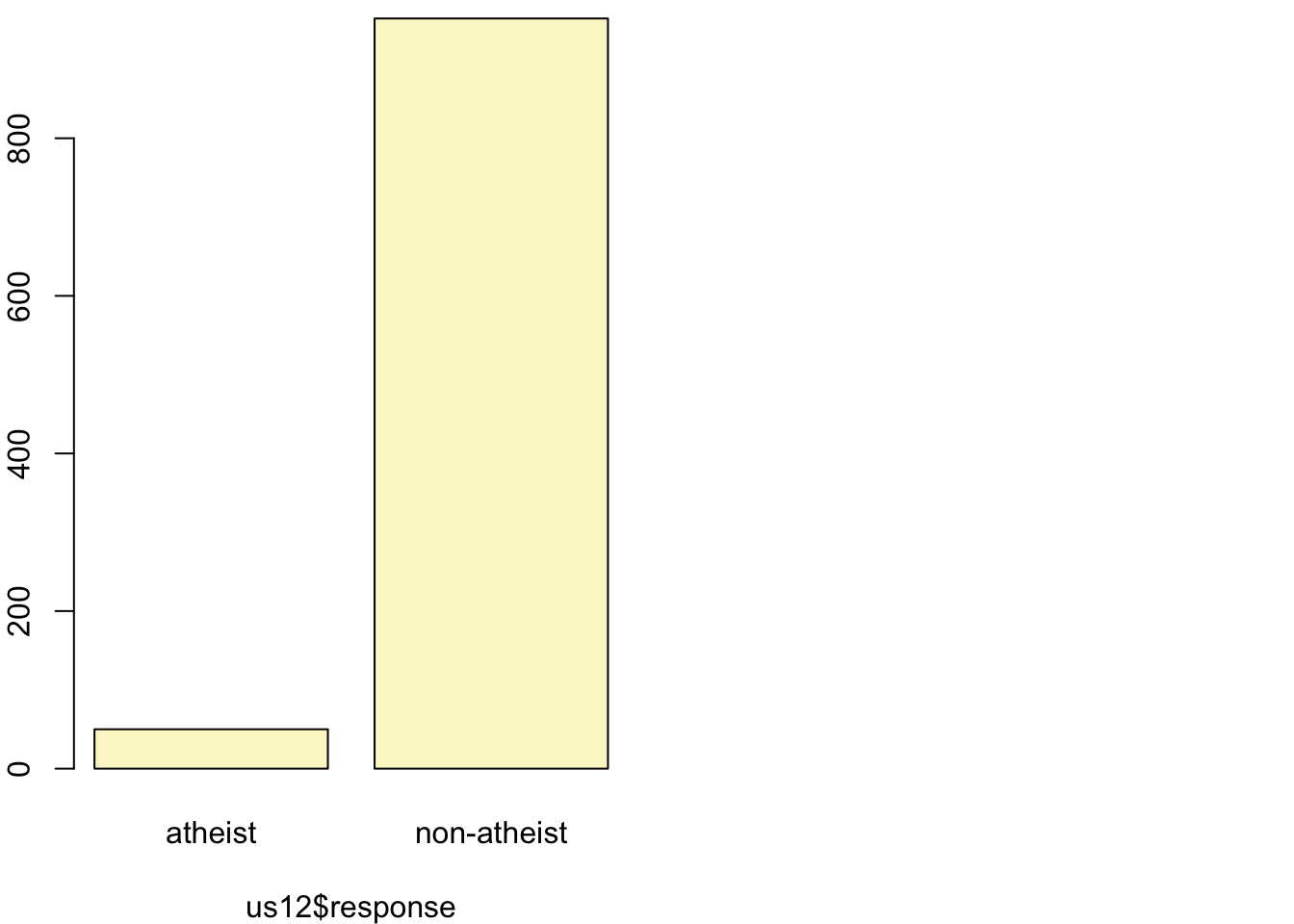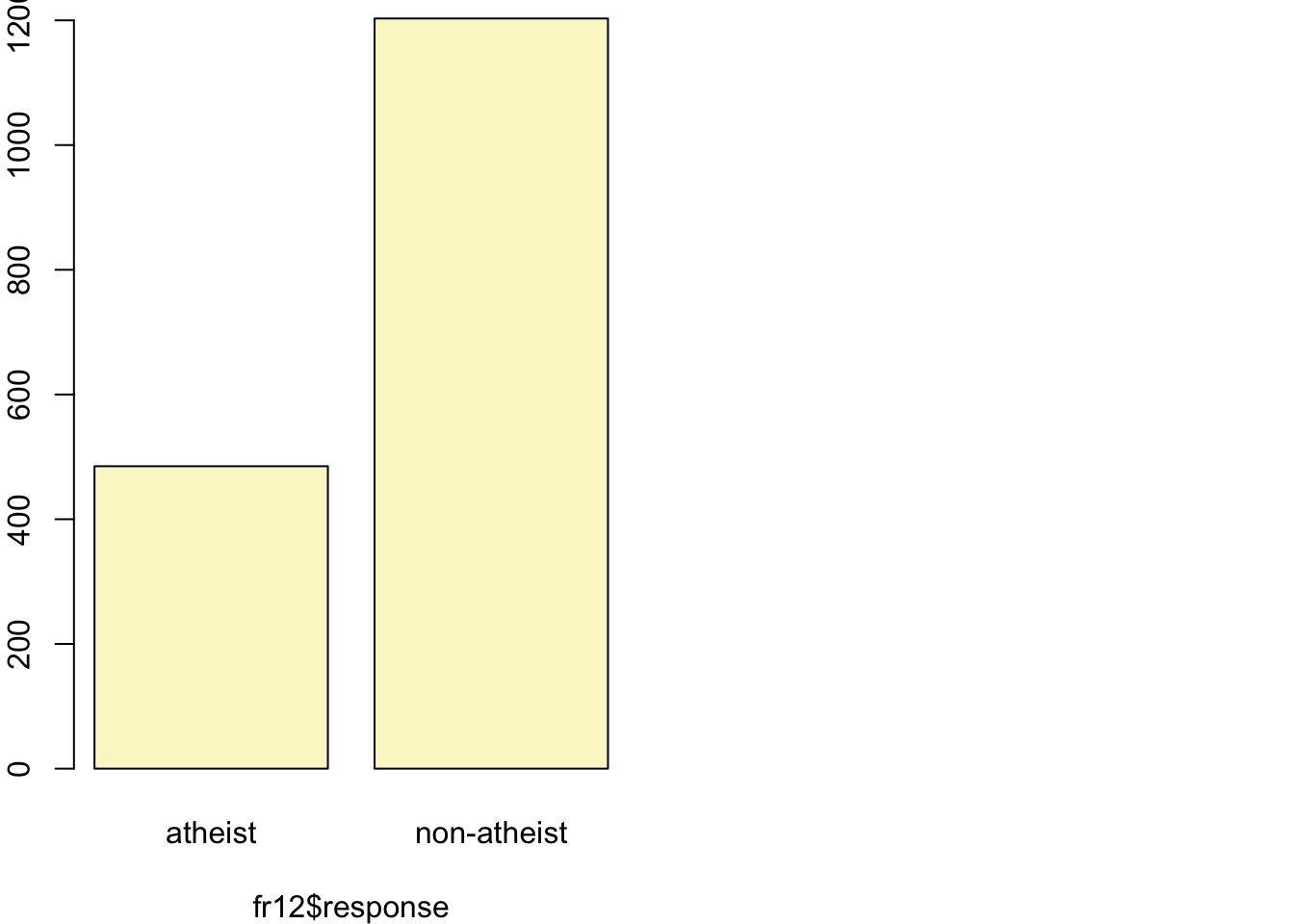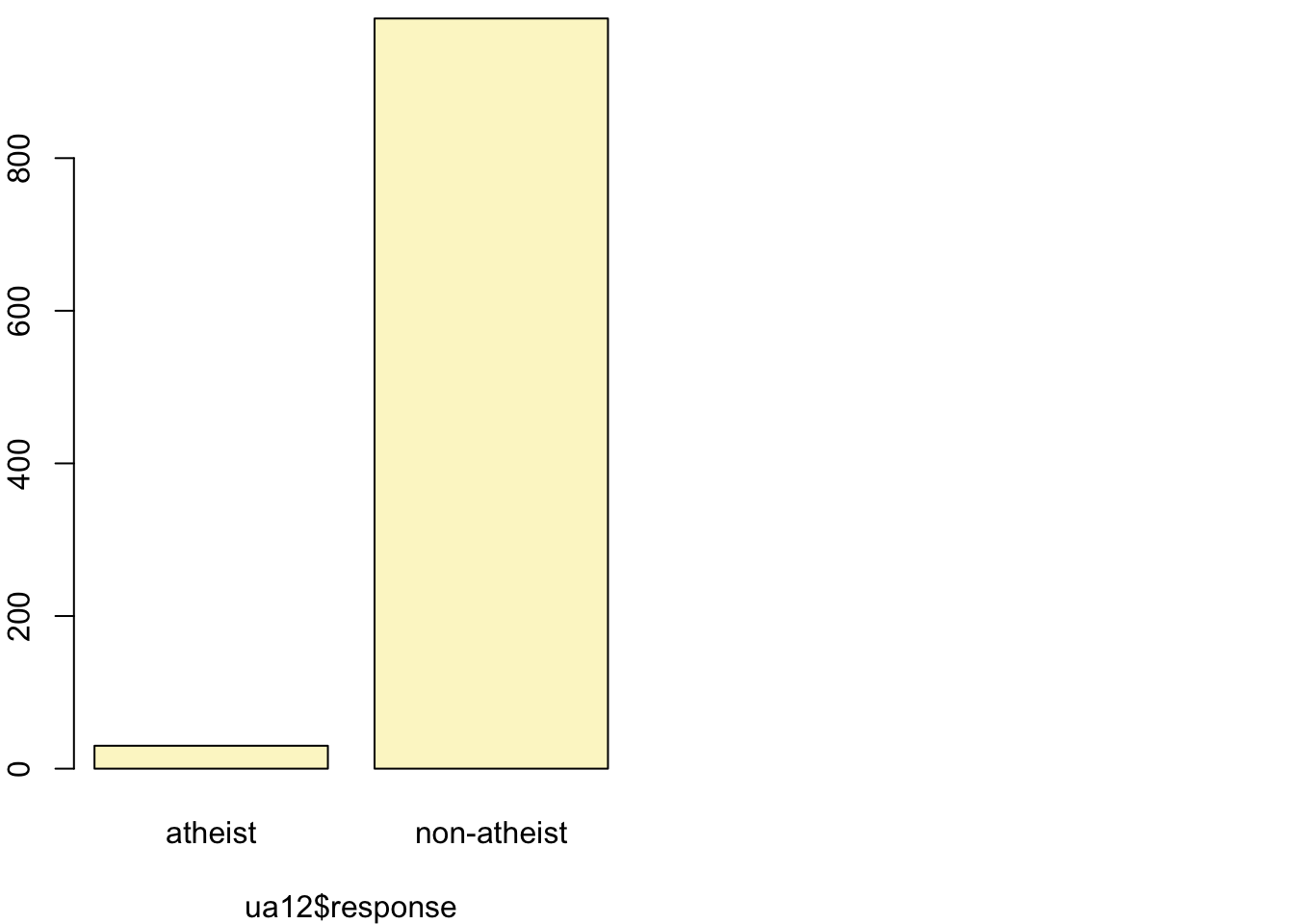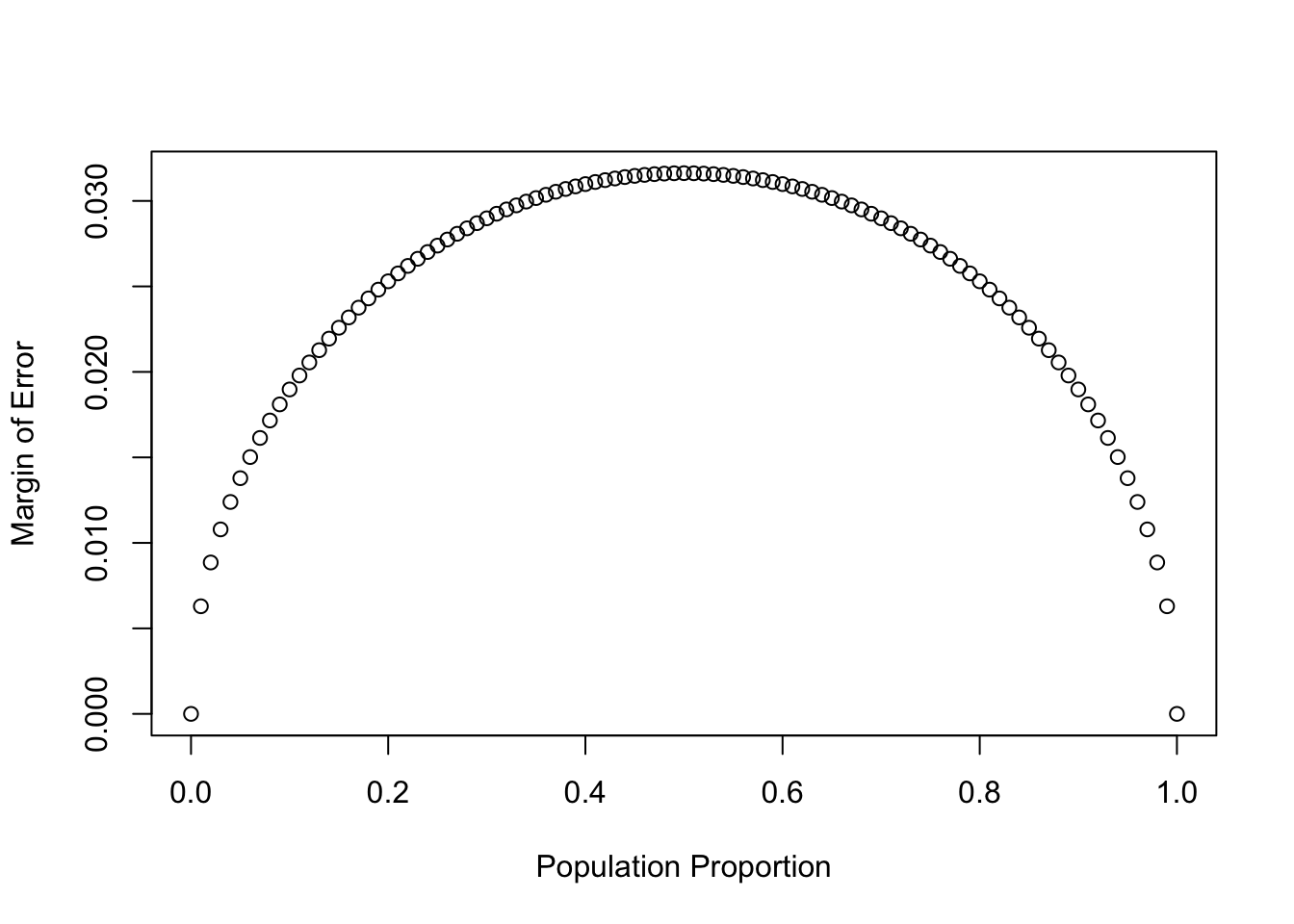#### December 2nd, 2018

In August of 2012, news outlets ranging from the Washington Post to the Huffington Post ran a story about the rise of atheism in America. The source for the story was a poll that asked people, “Irrespective of whether you attend a place of worship or not, would you say you are a religious person, not a religious person or a convinced atheist?” This type of question, which asks people to classify themselves in one way or another, is common in polling and generates categorical data. In this lab we take a look at the atheism survey and explore what’s at play when making inference about population proportions using categorical data.

## The survey

To access the press release for the poll, conducted by WIN-Gallup International, click on the following link:

https://github.com/jbryer/DATA606/blob/master/inst/labs/Lab6/more/Global_INDEX_of_Religiosity_and_Atheism_PR__6.pdf

Take a moment to review the report then address the following questions.

1. In the first paragraph, several key findings are reported. Do these percentages appear to be sample statistics (derived from the data sample) or population parameters?

These findings are sample statistics, based on the global poll.

2. The title of the report is “Global Index of Religiosity and Atheism”. To generalize the report’s findings to the global human population, what must we assume about the sampling method? Does that seem like a reasonable assumption?

• Independence, we will assume the sample is random and that observations are independent of each other.

• The sample must be less that 10% of the population. We can assume that condition is met since it is likely the poll did not sample 70,000,000 people.

## The data

Turn your attention to Table 6 (pages 15 and 16), which reports the sample size and response percentages for all 57 countries. While this is a useful format to summarize the data, we will base our analysis on the original data set of individual responses to the survey. Load this data set into R with the following command.

load("more/atheism.RData")
1. What does each row of Table 6 correspond to? What does each row of atheism correspond to?
summary(atheism)
##              nationality           response          year
##  Pakistan          : 5409   atheist    : 5498   Min.   :2005
##  France            : 3359   non-atheist:82534   1st Qu.:2005
##  Korea, Rep (South): 3047                       Median :2012
##  Ghana             : 2995                       Mean   :2009
##  Macedonia         : 2418                       3rd Qu.:2012
##  Peru              : 2414                       Max.   :2012
##  (Other)           :68390

Each row if table 6 corresponds to survey results for a population of a country.

Each row in “atheism” table corresponds to an individual person’s response to “atheist/non-atheist question only.

To investigate the link between these two ways of organizing this data, take a look at the estimated proportion of atheists in the United States. Towards the bottom of Table 6, we see that this is 5%. We should be able to come to the same number using the atheism data.

1. Using the command below, create a new dataframe called us12 that contains only the rows in atheism associated with respondents to the 2012 survey from the United States. Next, calculate the proportion of atheist responses. Does it agree with the percentage in Table 6? If not, why?
us12 <- subset(atheism, nationality == "United States" & year == "2012")

summary(us12)
##         nationality          response        year
##  United States:1002   atheist    : 50   Min.   :2012
##  Afghanistan  :   0   non-atheist:952   1st Qu.:2012
##  Argentina    :   0                     Median :2012
##  Armenia      :   0                     Mean   :2012
##  Australia    :   0                     3rd Qu.:2012
##  Austria      :   0                     Max.   :2012
##  (Other)      :   0
50/1002
##  0.0499002

Yes, our calculations show 5% which agrees with Table 6.

## Inference on proportions

As was hinted at in Exercise 1, Table 6 provides statistics, that is, calculations made from the sample of 51,927 people. What we’d like, though, is insight into the population parameters. You answer the question, “What proportion of people in your sample reported being atheists?” with a statistic; while the question “What proportion of people on earth would report being atheists” is answered with an estimate of the parameter.

The inferential tools for estimating population proportion are analogous to those used for means in the last chapter: the confidence interval and the hypothesis test.

1. Write out the conditions for inference to construct a 95% confidence interval for the proportion of atheists in the United States in 2012. Are you confident all conditions are met?
• The sample observations are independent. We can assume that the sample was randomly selected and we can be confident that the sample is < 10% of the population.
• We expected to see at least 10 successes and 10 failures in our sample (the success-failure condition). Our sample is large enough to meet this condition.

If the conditions for inference are reasonable, we can either calculate the standard error and construct the interval by hand, or allow the inference function to do it for us.

inference(us12$response, est = "proportion", type = "ci", method = "theoretical", success = "atheist") ## Single proportion -- success: atheist ## Summary statistics:## p_hat = 0.0499 ; n = 1002 ## Check conditions: number of successes = 50 ; number of failures = 952 ## Standard error = 0.0069 ## 95 % Confidence interval = ( 0.0364 , 0.0634 ) Note that since the goal is to construct an interval estimate for a proportion, it’s necessary to specify what constitutes a “success”, which here is a response of "atheist". Although formal confidence intervals and hypothesis tests don’t show up in the report, suggestions of inference appear at the bottom of page 7: “In general, the error margin for surveys of this kind is $$\pm$$ 3-5% at 95% confidence”. 1. Based on the R output, what is the margin of error for the estimate of the proportion of the proportion of atheists in US in 2012? #Margin of Error 1.96*0.0069 ##  0.013524 1. Using the inference function, calculate confidence intervals for the proportion of atheists in 2012 in two other countries of your choice, and report the associated margins of error. Be sure to note whether the conditions for inference are met. It may be helpful to create new data sets for each of the two countries first, and then use these data sets in the inference function to construct the confidence intervals. Calculating Confidence Interval and Margin of Error for France and Ukraine. #France fr12 <- subset(atheism, nationality == "France" & year == "2012") inference(fr12$response, est = "proportion", type = "ci", method = "theoretical",
success = "atheist")
## Single proportion -- success: atheist
## Summary statistics:## p_hat = 0.2873 ;  n = 1688
## Check conditions: number of successes = 485 ; number of failures = 1203
## Standard error = 0.011
## 95 % Confidence interval = ( 0.2657 , 0.3089 )
#Margin of Error
1.96*0.011
##  0.02156
summary(fr12$response) ## atheist non-atheist ## 485 1203 #Ukraine ua12 <- subset(atheism, nationality == "Ukraine" & year == "2012") inference(ua12$response, est = "proportion", type = "ci", method = "theoretical",
success = "atheist")
## Single proportion -- success: atheist
## Summary statistics:## p_hat = 0.0296 ;  n = 1013
## Check conditions: number of successes = 30 ; number of failures = 983
## Standard error = 0.0053
## 95 % Confidence interval = ( 0.0192 , 0.0401 )
#Margin of Error
1.96*0.0053
##  0.010388
summary(ua12\$response)
##     atheist non-atheist
##          30         983

The conditions for the inference are met since we assume the sample was randomly selected and the sample size are less than 10% of the total populations for these two countries. The success-failure conditon since there are more that 10 success/failures in each of those countries.

## How does the proportion affect the margin of error?

Imagine you’ve set out to survey 1000 people on two questions: are you female? and are you left-handed? Since both of these sample proportions were calculated from the same sample size, they should have the same margin of error, right? Wrong! While the margin of error does change with sample size, it is also affected by the proportion.

Think back to the formula for the standard error: $$SE = \sqrt{p(1-p)/n}$$. This is then used in the formula for the margin of error for a 95% confidence interval: $$ME = 1.96\times SE = 1.96\times\sqrt{p(1-p)/n}$$. Since the population proportion $$p$$ is in this $$ME$$ formula, it should make sense that the margin of error is in some way dependent on the population proportion. We can visualize this relationship by creating a plot of $$ME$$ vs. $$p$$.

The first step is to make a vector p that is a sequence from 0 to 1 with each number separated by 0.01. We can then create a vector of the margin of error (me) associated with each of these values of p using the familiar approximate formula ($$ME = 2 \times SE$$). Lastly, we plot the two vectors against each other to reveal their relationship.

n <- 1000
p <- seq(0, 1, 0.01)
me <- 2 * sqrt(p * (1 - p)/n)
plot(me ~ p, ylab = "Margin of Error", xlab = "Population Proportion")1. Describe the relationship between p and me.

For the population proportion between 0 and 0.5 there is a direct correlation, do the proportion between 0.5 and 1 - there is an inverse correlation.

## Success-failure condition

The textbook emphasizes that you must always check conditions before making inference. For inference on proportions, the sample proportion can be assumed to be nearly normal if it is based upon a random sample of independent observations and if both $$np \geq 10$$ and $$n(1 - p) \geq 10$$. This rule of thumb is easy enough to follow, but it makes one wonder: what’s so special about the number 10?

The short answer is: nothing. You could argue that we would be fine with 9 or that we really should be using 11. What is the “best” value for such a rule of thumb is, at least to some degree, arbitrary. However, when $$np$$ and $$n(1-p)$$ reaches 10 the sampling distribution is sufficiently normal to use confidence intervals and hypothesis tests that are based on that approximation.

We can investigate the interplay between $$n$$ and $$p$$ and the shape of the sampling distribution by using simulations. To start off, we simulate the process of drawing 5000 samples of size 1040 from a population with a true atheist proportion of 0.1. For each of the 5000 samples we compute $$\hat{p}$$ and then plot a histogram to visualize their distribution.

p <- 0.1
n <- 1040
p_hats <- rep(0, 5000)

for(i in 1:5000){
samp <- sample(c("atheist", "non_atheist"), n, replace = TRUE, prob = c(p, 1-p))
p_hats[i] <- sum(samp == "atheist")/n
}

hist(p_hats, main = "p = 0.1, n = 1040", xlim = c(0, 0.18))